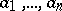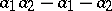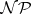# Frobenius problem

(diff) ← Older revision | Latest revision (diff) | Newer revision → (diff)

coin-change problem

Givennatural numberswith greatest common divisor, find the largest natural number that is not expressible as a linear non-negative integer combination of the.

Forthe answer is given by. The general problem is-hard.

For a fixedthere is a polynomial-time algorithm to solve the Frobenius problem, [a1].

The Frobenius problem is related to the study of maximal lattice-point-free convex bodies (in the geometry of numbers), [a2].

How to Cite This Entry:
Frobenius problem. Encyclopedia of Mathematics. URL: http://encyclopediaofmath.org/index.php?title=Frobenius_problem&oldid=16167
This article was adapted from an original article by M. Hazewinkel (originator), which appeared in Encyclopedia of Mathematics - ISBN 1402006098. See original article